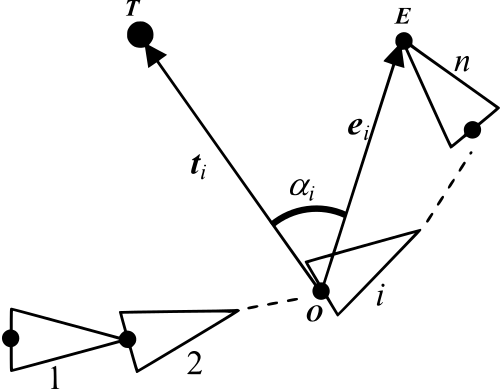# Cyclic Coordonate descent Inverse Kynematic (CCD IK)The goal of Inverse kinematic is simple, given a target point in space $$T$$ (blue cube) find the best rotation for each joint so that the tip of the last joint reaches the target. Many algorithms exist but the one presented below is called CCD IK. You can also constraint joints to rotate around specific axis (CCD+hinge) and also to only rotate within a certain range (CCD+hinge+range), you can test it out with the webgl snippet below:

Inspired by the blog post of Johnathon Selstad on CCD IK as an exercise for myself I wanted to re-word the post and implement my own shader toy version.

### Summary of the simple CCD Inverse Kynematic algorithm

• $$T$$ : Position of the target
• $$E$$ : Position of the end-effector (tip of the last joint)
• $$O$$ : Pivot point of the ith link, $$i=1, 2, …, n$$
• $$t_i$$: Target vector for the ith link = $$T - O_i$$
• $$e_i$$ : End-effector vector for the ith link = $$E − O_i$$
• $$\alpha_i$$ : Angle between vectors $$t_i$$ and $$v_i$$ .The core of the CCD IK algorithm is extremely simple:

for( r = 0; r < nb_iterations; r++){
// Iterate from tip to base
for( i = n; i > 0; i++){
rotation_i = rotation_between(e_i, t_i)
// rotate ith link by alpha_i so that the end-effector meets the target vector ti
joint[i].rotate_by( rotation_i )
}
}


Constraining the rotation to a specific axis is quite simple as well. Let $$axis_i$$ be the current axis, given the ith CCD rotation found in the first step we rotate $$axis_i$$ which gives us the new axis $$axis_i'$$. We just rotate back the ith joint by the rotation defined between $$axis_i$$ and $$axis_i'$$:

for( r = 0; r < nb_iterations; r++){
for( i = n; i > 0; i++){
rotation_i = rotation_between(e_i, t_i)
joint[i].rotate_by( rotation_i )

current_axis = rotation_i * joint[i].axis;
rotation_back = rotation_between( current_axis, joint[i].axis )

joint[i].rotate_by( rotation_back )

}
}


Now we can constrain the range of the rotation as well. Wether your rotations are represented by a Quaternion or a Matrix you can extract the pair (axis, angle), clamp the angle and convert back to the final matrix/quaternion:

for( r = 0; r < nb_iterations; r++){
for( i = n; i > 0; i++){
rotation_i = rotation_between(e_i, t_i)
joint[i].rotate_by( rotation_i )

current_axis = rotation_i * joint[i].axis;
rotation_back = rotation_between( current_axis, joint[i].axis )

joint[i].rotate_by( rotation_back )
(axis, angle) = extract_axis_angle( joint[i].get_rotation() )
joint[i].set_rotation( build_rotation(axis, clamp(angle, min, max)) )
}
}